# Christoffel Symbols in terms of a Change in Basis

• A
Phinrich
TL;DR Summary
Can we express thew Christoffel Symbols in terms of the change of basis?
Hi All

Given that the Riemann Curvature Tensor may be derived from the parallel Transport of a Vector around a closed loop, and if that vector is a covariant vector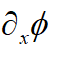Having contravariant basis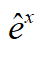The calculation gives the result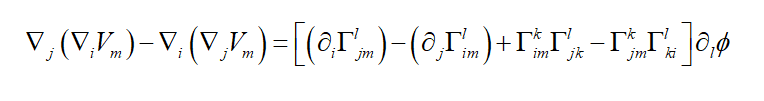Now:

Given that the Christoffel Symbols represent the change in the basis vectors (rotations etc) as we move around the closed loop and given that mathematically this is given by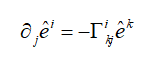Can we represent the Riemann Curvature Tensor in terms of the basis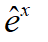Thank You

#### Attachments

kent davidge
Rule of thumb is if your tensor has one or more lower indices, then it cannot be written only in terms of the contravariant basis. In the case of the Riemann tensor, it goes like ##R = R^\mu{}_{\nu \sigma \rho} dx^\nu dx^\sigma dx^\rho e_\mu##.

kent davidge
if that vector is a covariant vector

View attachment 251477

Having contravariant basis

View attachment 251478
if it is a covariant vector it cannot have a contravariant basis

Jianbing_Shao
The problem is if the curvatrue is not 0, then obviously we can not define a gobal basis vector ##e_\mu(x)## on the manifold.
but locally the change of basis vectors can be described using a matrix function ##A(x)## , then the connection can be expressed in such a way:
##\Gamma_i(x)\doteq \partial_i A(x)A^{-1}(x) ##

Phinrich
Rule of thumb is if your tensor has one or more lower indices, then it cannot be written only in terms of the contravariant basis. In the case of the Riemann tensor, it goes like ##R = R^\mu{}_{\nu \sigma \rho} dx^\nu dx^\sigma dx^\rho e_\mu##.

I agree that in the case of both upstairs and downstairs indices on the Riemann Curvature Tensor it cannot be written only in terms of contravarient basis. What if I was looking at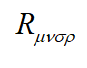Phinrich
The problem is if the curvatrue is not 0, then obviously we can not define a gobal basis vector ##e_\mu(x)## on the manifold.
but locally the change of basis vectors can be described using a matrix function ##A(x)## , then the connection can be expressed in such a way:
##\Gamma_i(x)\doteq \partial_i A(x)A^{-1}(x) ##

I agree that we can only express a basis locally. Please could you elaborate on the Equation involving A(x) and can the A(x) be written in terms of or related to the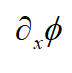or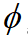of the vector transported ?

Thanks

Phinrich

I agree that we can only express a basis locally. Please could you elaborate on the Equation involving A(x) and can the A(x) be written in terms of or related to the View attachment 251527 or View attachment 251528 of the vector transported ?

Thanks
OK

In a 3-space I think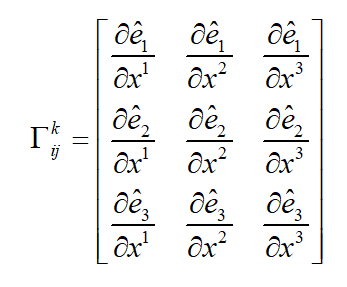Gold Member
2022 Award
$$\newcommand{\uvec}{\underline{#1}}$$
if it is a covariant vector it cannot have a contravariant basis
You have to distinguish between vectors/tensors and their components. A vector or tensor is an invariant object, i.e., it doesn't depend on the choice of the basis.

A vector is decomposed into components ##V^{j}## with respect to a basis ##\vec{b}_j##,
$$\vec{V}=V^{j} \vec{b}_j.$$
Here and in the following the Einstein summation convention applies.

Now changing the basis to ##\vec{b}_j'## via
$$\vec{b}_j'={T^k}_j \vec{b}_k,$$
which by definition is the covariant transformation law. It's easy to derive how the vector components change:
$$\vec{V}=V^{\prime j} \vec{b}_j'=V^{\prime j} {T^k}_j \vec{e}_k=V^k \vec{e}_k,$$
i.e.
$$V^k={T^k}_j V^{\prime j}$$
or introducing the inverse ##{U^j}_k## of the matrix ##{T^k}_j##, i.e., ##{U^{j}}_k {T^k}_l=\delta_l^k##:
$$V^{\prime j}={U^j}_k T^{k}.$$
This is the contravariant transformation law. One also says that the components transform contragrediently to the basis vectors such that the vector stays invariant.

Now there are also the dual vectors, i.e., the linear forms. Given a basis ##\vec{b}_j## for the vectors there's a corresponding dual basis ##\underline{b}^k## defined as the linear form mapping the basis vectors as
$$\uvec{b}^k(\vec{b}_j)=\delta_j^k.$$
An arbitrary linear form is then given by
$$\uvec{L}=L_j \uvec{b}^j.$$
Now we have
$$\uvec{L}(\vec{V})=L_j \uvec{b}^j(V^k \vec{b}_k)=L_j V^k \delta_k^j = L_j V^j.$$
The same should hold true for the components in the other basis and its dual basis, i.e.,
$$\uvec{L}(\vec{V})=L_j' V^{\prime j}=L_j' {U^j}_k V^k=L_k V^k,$$
i.e.,
$$L_k={U^j}_k L_j' \; \Leftrightarrow \; L_j'={T^k}_j L_k.$$
The components of a linear form thus transform covariantly. From this we also get
$$\uvec{L}=L_k \uvec{b}^k = {U^j}_k L_j' \uvec{b}^k=L_j' \uvec{b}^{\prime j},$$
i.e.,
$$\uvec{b}^{\prime j}={U^j}_k \uvec{b}^k,$$
i.e., the dual basis vectors transform contravariantly, and thus the linear form is indeed invariant, as it must be:
$$\uvec{L}=L_j \uvec{b}^j=L_k' \uvec{b}^{\prime k}.$$

•pervect
Phinrich
Thank you Kent Davidge.

Your explanation is very clear indeed.

However I am not referring to instances where we remain at the same local region, in space, and simply change our basis and ask how does the tensor transform to a new basis. Imagine a global manifold and we are in a local region. Now we move to a second local region and ask how will our basis vectors rotate/move to the new local region? Is this not what the Christoffel symbols represent? How may I express these symbols in terms of the basis of the gradient tensor (if at all possible)?

Thanks

Gold Member
2022 Award
As I said, a tensor doesn't transform when you change the basis. It's an invariant basis-independent mathematical object. What transforms are the bases and the components of tensor with respect to these bases.

The Christoffel symbols are components of a socalled connection, which allows you to transport (again in a basis and coordinate-independent way) the tangent vector on a manifold at one point to the tangent space at another place. Of course you can transport also a basis of the tangent space at one point to another point, leading to a basis of the tangent space at this point.

Staff Emeritus
I agree with vanhees's points. I'd suggest re-writing (if possible) the original question taking into mind these points. And there are a few other things to clean up along the way. These points seem minor, but they reflect some errors in communication at minimum, and possibly some more significant errors.

original poster said:
Given that the Riemann Curvature Tensor may be derived from the parallel Transport of a Vector around a closed loop, and if that vector is a covariant vector

Here the OP writes for some reason ##\partial_x \phi## , but I think it should be just ##\partial_x##. The partial derivative operator is a vector, it doesn't take an argument ##\phi##. More on this later.

OP said:
Having contravariant basis ##\hat{e}^x##

But the contravariant basis is ##e_j##. There may or may not be some significance in using "j" rather than "x", the primary point is, as vanhees explained earlier, that a vector \vec{v} is

$$\vec{v} = \sum_{j} v^j \, \vec{e}_j$$

So ##\vec{v}## here is the geometric object, the vector, which are interpreted to "exist" independently of any basis, and the ##v^j## are the components of the vectors in some specific basis. Each of the ##\vec{e}_j## is a vector, j being a selector rather than an index. So we have ##\vec{e}_0, \vec{e}_1, \vec{e}_2, \vec{e}_3## as a set of four basis vectors, and in ##\vec{e}_j## the j selects which member of this set of vectors we are picking out.

The above is very general - we are just saying an arbitrary vector can be expressed as weighted sum of basis vectors. But let's talk now about a special basis, the coordinate basis.

When we introduce coordinates ##x^i##, we also introduce a set of basis vectors associated with those coordinates called the coordinate basis. Formally, these are identified as ##\vec{e}_i = \frac{\partial}{\partial x^i}##, i.e. the coordinate basis vectors are just partial derivative operators.

Let's skip on a bit, and get to where things start to get really confused

OP said:
Given that the Christoffel Symbols represent the change in the basis vectors (rotations etc) as we move around the closed loop and given that mathematically this is given by

On a manifold, every point p on that manifold has it's own set of basis vectors. The process of "moving around the closed loop" is formally called a process of parallel transport. So we can say that when we parallel transport a vector around a closed loop, the vector itself is changed by this transport process.

An example here might help, such as http://math.ucr.edu/home/baez/gr/parallel.transport.html

What the Christoffel symbols actually do is tell you how, in a coordinate basis, to parallel transport a vector from one point p to a nearby point p'. So it provides a "connection".

I'd write out how this is done in more detail, but this post is already too long, and the OP would probably be better served by reading about it in whatever textbook he uses. My general thoughts are there are a lot of little niggling details that are getting in the way of communication, for instance the idea that vectors are just partial derivative operators. More significantly, I think the whole concept of "parallel transport" has gotten lost (or perhaps never been found) by the original poster, and that it would be a good thing for them to study it more. I would suggest doing something like Baez's example, and consider the process of parallel transporting a vector along a sphere to get a better mental picture of just what this involves.

The geometric construction known as "Schild's ladder" is a personal favorite of mine as far as parallel transport goes. However, it's a bit quirky. I wouldn't mind going into more details if it'd help the OP understand parallel transport, but I'm concerned that it might just confuse them further. I suppose I can say that if one already knows how to draw geodesic curves (for instance, one knows that great circles are geodesics on the 2-sphere), one can use this geometric technique to carry out the parallel transport operation, and show by example that vectors actually change (rotate) when they're parallel transported along a closed loop on a sphere, while they do no such thing on a plane.

Jianbing_Shao

I agree that we can only express a basis locally. Please could you elaborate on the Equation involving A(x) and can the A(x) be written in terms of or related to the View attachment 251527 or View attachment 251528 of the vector transported ?

Thanks
If ##\phi## is a scalar field, so the vector field ## \partial_i\phi## is a gradient field , then the curl of the vector field is 0, But to an arbitrary vector field ##v_i(x)##, the curl is not always 0, So to an arbitrary vector field, it usually can not be expressed using a scalar field ##\phi(x)##.

Further more, if the curvature of a manifold is not 0, then the parallel transport of a vector on the manifold around a closed path will cause a change of the vector, so we can assert that it is impossible to get a vector field on the manifold, just as we can not define a globle vector basis on the manifold.

So I an afraid the Riemann curvature tensor can not be described using ##\partial_i\phi(x)## or ##\phi(x)##

Phinrich
Thank you everyone for your helpful comments and suggestions. I will study them all carefully to improve my understanding of this.

Gold Member
2022 Award
But the contravariant basis is ##e_j##. There may or may not be some significance in using "j" rather than "x", the primary point is, as vanhees explained earlier, that a vector \vec{v} is

$$\vec{v} = \sum_{j} v^j \, \vec{e}_j$$
The nomenclature, at least in physics, is different. One calls objects with a lower index "covariant" and an object with an upper index "contravariant", i.e., in this case of a vector its components ##v^j## transform contravariantly and the basis vectors ##\vec{e}_j## transform covariantly. See #8 for the details.

dsaun777
OK

In a 3-space I think

View attachment 251529
aren't you missing an extra term ek basis term on the right?

Phinrich
Thank you. Thats
aren't you missing an extra term ek basis term on the right?
a neat way of putting it.

Paul

dsaun777
As I said, a tensor doesn't transform when you change the basis. It's an invariant basis-independent mathematical object. What transforms are the bases and the components of tensor with respect to these bases.

The Christoffel symbols are components of a socalled connection, which allows you to transport (again in a basis and coordinate-independent way) the tangent vector on a manifold at one point to the tangent space at another place. Of course you can transport also a basis of the tangent space at one point to another point, leading to a basis of the tangent space at this point.
Invariants are built out of components and bases, but aren't the bases "vectors" themselves and should be considered invariant?

Mentor
aren't the bases "vectors" themselves and should be considered invariant?

You have to be very careful about what "invariant" means.

If we are talking about vectors at a single point, yes, those are invariant (more precisely, they are covariant, which means expressions involving them that have no free indexes are invariants).

But if we are talking about Christoffel symbols, we are talking about how to transport vectors (and tensors and other geometric objects) from one point to another. This process of transport can change the vectors. The vectors we call "basis vectors" are just as subject to being changed by the transport process as any others. In fact, the connection is what tells us how all vectors (and tensors and other geometric objects), including the basis vectors, change as a result of the transport process.

•dsaun777
dsaun777
Why is it, in relativity, that the christoffel symbols are almost always defined with the permutation of the metric derivatives instead of just the more straightforward change of basis?

Gold Member
2022 Award
Invariants are built out of components and bases, but aren't the bases "vectors" themselves and should be considered invariant?
Of course each basis is a set of invariant vectors, but if you change the basis you change it, right?

As an example take a Euclidean ##\mathbb{R}^2## and the change from a Cartesian basis to standard polar-coordinate basis (not holonomous but the usual orthonormal basis). The Cartesian basis is convenient, because the basis is constant over the entire plane. Let's call this basis ##\vec{e}_i## (##i \in \{1,2 \}##) and the bases of polar coordinates, which are dependent on the point on the plane ##\vec{e}_i'(r,\vartheta)##. For the position vector we write ##\vec{r}=x^i \vec{e}_i = x \vec{e}_1 + y \vec{e}_2##. Then for any vector field you have
$$\vec{V}(\vec{r})=\vec{e}_i V^i(x,y) = \vec{e}_j'(r,\vartheta) V^{\prime j}(r, \vartheta).$$
To get the components of the position vector in the polar-coordinate basis we note that
$$x=r \cos \vartheta, \quad y=r \sin \vartheta$$
and thus
$$\partial_r \vec{r}=\cos \vartheta \vec{e}_1 + \sin \vartheta \vec{e}_2 \; \Rightarrow \; g_r=|\partial_{r} \vec{r}|=1$$
and
$$\partial_{\vartheta} \vec{r}=-r \sin \vartheta \vec{e}_1 + r \cos \vartheta \vec{e}_2 \; \Rightarrow\; g_{\vartheta}=|\partial_{\vartheta} \vec{r}|=r.$$
Thus we have
$$\vec{e}_1'(r,\vartheta)=\frac{1}{g_r} \partial_r \vec{r}=\cos \vartheta \vec{e}_1 + \sin \vartheta \vec{e}_2, \quad \vec{e}_2'(r,\vartheta)=\frac{1}{g_\vartheta} \partial_{\vartheta} \vec{r} = -\sin \vartheta \vec{e}_1 + \cos \vartheta \vec{e}_2.$$
Now we have
$$\vec{e}_j'(r,\vartheta)=D_{ij}(r,\vartheta) \vec{e}_i$$
with the transformation matrix (which is here of course orthogonal, because we change between orthonormal bases)
$$D_{ij}(r,\vartheta)=\vec{e}_i \cdot \vec{e}_j' \; \Rightarrow \; \hat{D}=\begin{pmatrix} \cos \vartheta & -\sin \vartheta \\ \sin \vartheta & \cos \vartheta \end{pmatrix}.$$
The transformation of the vector components thus follows from
$$V^{\prime j} \vec{e}_j' = V^{\prime j} D_{ij} \vec{e}_i \; \Rightarrow \; V^{i}=D_{ij} V^{\prime j}.$$
Now it's easy to derive the usual vector operators on scalar and vector-field components. So let ##\Phi## be a scalar field. Then the gradient is defined by
$$\mathrm{d} \Phi=\mathrm{d} x^i \partial_i \Phi:=\mathrm{d} \vec{r} \cdot \vec{\nabla} \Phi.$$
Since this is still a scalar, ##\vec{\nabla} \Phi## must be a vector field. Denoting ##q^1=r## and ##q^2=\vartheta## we have
$$\mathrm{d} \Phi=\mathrm{d} q^j \frac{\partial}{\partial q^j} \Phi = \mathrm{d} q^j \frac{\partial \vec{r}}{\partial q^j} \cdot \vec{\nabla} \Phi.$$
$$\mathrm{d} \Phi=(\mathrm{d} r g_r \vec{e}_1' + \mathrm{d} \vartheta g_{\vartheta} \vec{e}_2') \cdot \vec{\nabla} \Phi=\mathrm{d}_r \frac{\partial \Phi}{\partial r} + \mathrm{d} \vartheta \frac{\partial \Phi}{\partial \vartheta}$$
and thus
$$(\nabla \Phi)^{'1}=\frac{1}{g_r} \partial_r \Phi=\partial_r \Phi, \quad (\nabla \Phi)^{'2}=\frac{1}{g_{\vartheta}} \partial_{\vartheta} \Phi=\frac{1}{r} \partial_{\vartheta} \Phi.$$
For vector fields one has to be careful to take into account the dependence of the basis vector on the generalized coordinates. The most elegant method to get the expressions of the operators ##\text{div}## and ##\text{curl}## is to use their coordinate independent definitions through integrals. Another way is to use the simple expressions in Cartesian coordinates and transform them appropriately. For the divergence of a vector field we have
$$\mathrm{div} \vec{V}=\vec{\nabla} \cdot \vec{V}=\frac{\partial}{\partial x^i} V^i = \frac{\partial}{\partial x^i} D_{ij} V^{\prime j}=\frac{\partial q^k}{\partial x_i} \frac{\partial}{\partial q_k} D_{ij} V^{\prime j}.$$
Now we need the Hesse matrix
$$H_{ki}=\frac{\partial q^k}{\partial x_i}$$
which is inconvenient to calculate. We rather use its inverse defining
$$\tilde{H}_{ab}=\frac{\partial x^a}{\partial q^b}.$$
Indeed from
$$\frac{\partial x^a}{\partial x_b}=\delta_{ab} = \frac{\partial x_a}{\partial q_c} \frac{\partial q_c}{\partial x_b} = \tilde{H}_{ac} H_{cb}$$
we see that in matrix notation ##H=\tilde{H}^{-1}##. Now
$$\tilde{H}=\begin{pmatrix}\cos \vartheta & -r \sin \vartheta \\ \sin \vartheta & r \cos \vartheta\end{pmatrix}.$$
Then
$$H=\tilde{H}^{-1} =\frac{1}{r} \begin{pmatrix} r \cos \vartheta &r \sin \vartheta \\ -\sin \vartheta & \cos \vartheta \end{pmatrix}.$$
After some algebra one finally gets
$$\text{div} \vec{V}=\frac{1}{r} \partial_r (r V^{\prime 1}) + \frac{1}{r} \partial_{\vartheta} V^{\prime 2}.$$
The curl can be calculated in a similar way, though it's even more cumbersome ;-)).

•dsaun777